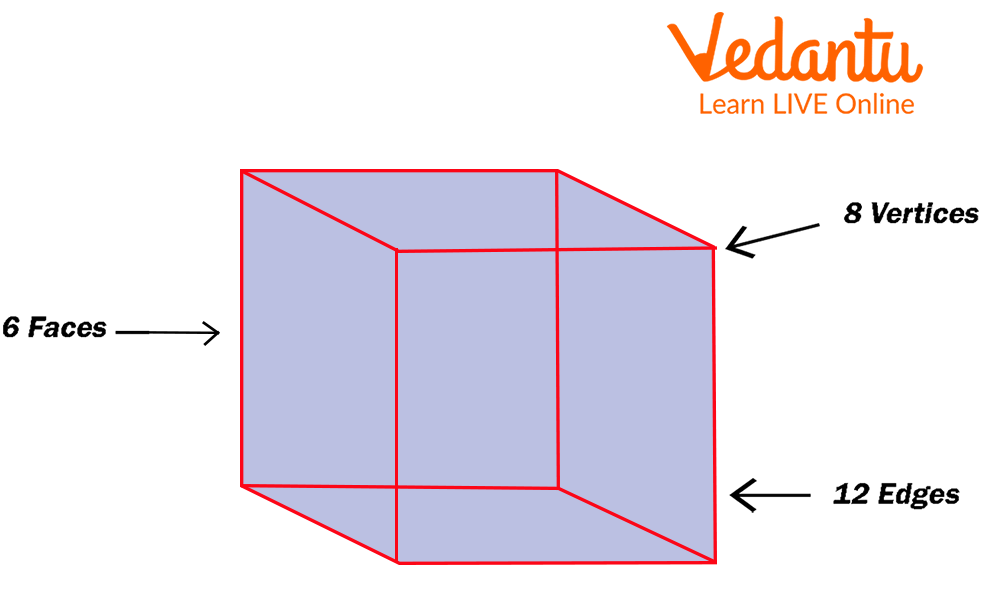Courses
Courses for Kids
Free study material
Free LIVE classes
MoreLIVE
Join Vedantu’s FREE Mastercalss

Maths is one of the unique languages of science that deals with numbers and builds logical thinking and analytical reasoning skills. So, Maths vocabulary plays a very crucial role in understanding the Maths concepts better and developing Maths skills. Many times, we see that students are unable to process or make sense of what they are doing. If a student is unable to develop a Maths concept fully, then it will lead to a misconception and lack of understanding of the next skills because this is a building-block process. Therefore, students should have these foundational building blocks to advance to higher levels.

## List of Mathematical Words Starting with the Letter ‘E’

In this section, we have explained some of the Mathematical terms that start with ‘e’ in detail with examples. So, try to explore all the words and save as much as possible in your vocabulary bank.

### Edge

In Geometry, an edge is a line segment that joins one vertex to another.

Example: A cube has 12 edges.Cube

### Endpoints

In Geometry, the points on either end of a line segment or one end of a ray are called endpoints.

Example:Endpoints

### Even Numbers

An even number is a number that can be divisible by 2 and after dividing even numbers by 2, we get 0 as a remainder. So, we can say that the numbers ending with 0, 2, 4, 6, and 8 are even numbers.

Example:

 List of Even Numbers from 1 to 100 2 4 6 8 10 12 14 16 18 20 22 24 26 28 30 32 34 36 38 40 42 44 46 48 50 52 54 56 58 60 62 64 66 68 70 72 74 76 78 80 82 84 86 88 90 92 94 96 98 100

## Equilateral Triangle

In Geometry, an equilateral triangle is a triangle that is composed of three sides equal in length and all the angles are equal in measure in an equilateral triangle.

Example:Equilateral Triangle

## Equivalent Fractions

In Mathematics, equivalent fractions can be defined as the fractions that might have different denominators and numerators but are equal to the same value. All equivalent fractions result in the same fraction in their simplest form.

Example: 1/4, 2/8, 4/16, 8/32 — all the fractions represent the same value that is 1/4. So, all these are equivalent fractions.

### Equation

In Maths, an equation is defined as a Mathematical statement that is composed of two algebraic expressions with the same value connected by an equal sign.

The algebraic expressions in Maths can have one or more than one variable.

Example: 5x + 10y = 20 is an equation where 5x + 10y and 20 are two expressions, connecting by an equal sign.

## Element of a Set

All the objects or items present in a set are called elements. All the elements in a set are separated by commas and enclosed by curly brackets.

Example: A={2, 4, 6, 8, 10} is a set where 2, 4, 6, 8, 10 are the elements of the set A.

## Empty Set

An empty set can be defined as a set that has no elements. It is also called a null set and is represented as {} or it can also be represented by using the symbol '∅'.

## Exponent

An exponent can be defined as a number that tells you how many times a number is multiplied by itself. Exponent is also represented as the power of a number.

Example: 4×4×4 is the expanded form of 43 where 4 is multiplied by itself three times. Here, ‘3’ is called the exponent or power and ‘4’ is called the base.

## Expanded Form

Expanded form is a way of showing up a number as a sum of each digit multiplied by its place value.

Example: 7892 can be expanded as 7000+800+90+2.

## Conclusion

In this article, we have covered the most important Mathematical terms with examples which start with the letter ‘e’. Students need to practise and learn these concepts so that they don’t face any difficulties while solving problems at higher levels.

Last updated date: 04th Oct 2023
Total views: 136.2k
Views today: 2.36k